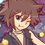# Enormous Harmonic Sum proof (help mehhh)

My friend asked me a question about this (from Russia IMO or something I forgot). The question asked for $n=2013$. I know the answer by just trying small numbers and then predict. Here's the question to prove:

Prove that $\sum\limits_{j=1}^n (\sum\limits_{i=j}^n \frac{1}{i})^{2} + \sum\limits_{i=1}^n \frac{1}{i} = 2n$

If you're confused with these stuffs, it is...

$(\frac{1}{1} + \frac{1}{2} + ... + \frac{1}{n})^{2} + (\frac{1}{2} + ... + \frac{1}{n})^{2} + ....... + (\frac{1}{n})^{2} + \frac{1}{1} + \frac{1}{2} + ... + \frac{1}{n} = 2n$Note by Samuraiwarm Tsunayoshi
7 years, 2 months ago

This discussion board is a place to discuss our Daily Challenges and the math and science related to those challenges. Explanations are more than just a solution — they should explain the steps and thinking strategies that you used to obtain the solution. Comments should further the discussion of math and science.

When posting on Brilliant:

• Use the emojis to react to an explanation, whether you're congratulating a job well done , or just really confused .
• Ask specific questions about the challenge or the steps in somebody's explanation. Well-posed questions can add a lot to the discussion, but posting "I don't understand!" doesn't help anyone.
• Try to contribute something new to the discussion, whether it is an extension, generalization or other idea related to the challenge.

MarkdownAppears as
*italics* or _italics_ italics
**bold** or __bold__ bold
- bulleted- list
• bulleted
• list
1. numbered2. list
1. numbered
2. list
Note: you must add a full line of space before and after lists for them to show up correctly
paragraph 1paragraph 2

paragraph 1

paragraph 2

[example link](https://brilliant.org)example link
> This is a quote
This is a quote
    # I indented these lines
# 4 spaces, and now they show
# up as a code block.

print "hello world"
# I indented these lines
# 4 spaces, and now they show
# up as a code block.

print "hello world"
MathAppears as
Remember to wrap math in $$ ... $$ or $ ... $ to ensure proper formatting.
2 \times 3 $2 \times 3$
2^{34} $2^{34}$
a_{i-1} $a_{i-1}$
\frac{2}{3} $\frac{2}{3}$
\sqrt{2} $\sqrt{2}$
\sum_{i=1}^3 $\sum_{i=1}^3$
\sin \theta $\sin \theta$
\boxed{123} $\boxed{123}$

Sort by:

This is from IMC 2013. :)

- 7 years, 2 months ago

Thanks! ^__^

- 7 years, 2 months ago

Let $H_n = \sum_{i=1}^n \frac1{i}$ (with the convention that $H_0 = 0$ ). My approach was to write the quantity on the left side as $S_n = \sum_{j=1}^n (H_n - H_{j-1})^2 + H_n$. It is enough to show that $S_{n+1}-S_n = 2$, since $S_1 = 2$ is the base case for an easy induction.

This difference equals $\sum_{j=1}^{n+1} \left( (H_{n+1}-H_{j-1})^2-(H_n-H_{j-1})^2 \right) + \frac1{n+1}$, which simplifies by a difference of squares formula to $\sum_{j=1}^{n+1} \frac1{n+1} ( H_{n+1} + H_n - 2 H_{j-1}) + \frac1{n+1}$, which becomes $H_{n+1} + H_n + \frac1{n+1} - \frac2{n+1} \sum_{j=1}^{n+1} H_{j-1} = 2 H_{n+1} - \frac2{n+1} \sum_{j=1}^{n+1} H_{j-1}$.

Ah, but now we can use the identity $\sum_{i=0}^n H_i = (n+1)H_n - n$, which is easy to prove by induction. (I found it on the wikipedia page for "harmonic number.") Plugging this in, we get $2 H_{n+1} - \frac2{n+1} ((n+1) H_n - n) = 2 H_{n+1} - 2 H_n + \frac{2n}{n+1} = \frac2{n+1} + \frac{2n}{n+1} = 2$ as desired.

There is probably a much more elegant way to do the problem (i.e. directly without induction), but this certainly works.

- 7 years, 2 months ago

Thank you for the proof. ^__^

- 7 years, 2 months ago

I don't quite understand how it is enough to prove that $S_{n+1} - S_{n} = 2$. I understand the rest but I'm just stuck at the first part. =_=" Sorry about that *cries

- 7 years, 2 months ago

$S_{n+1} - S_{n} = 2(n+1) - 2n = 2$

- 7 years, 2 months ago